# Label propagation - Semisupervised Learning with Applications to NLP

Jan. 24, 2013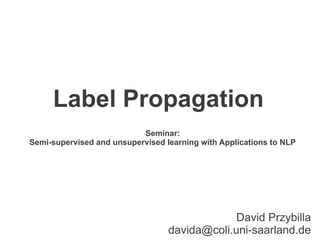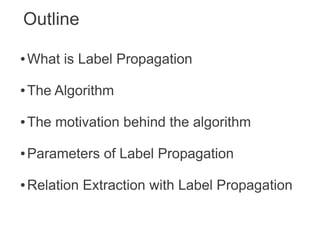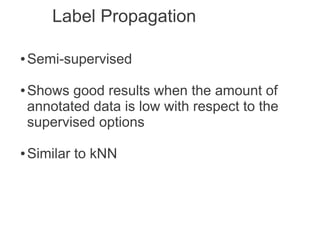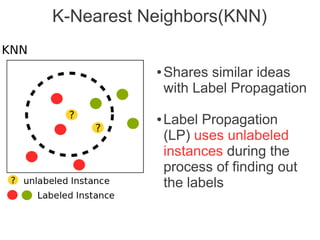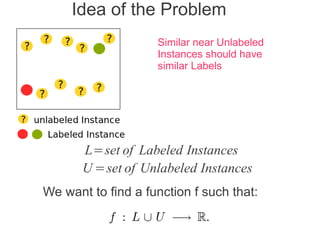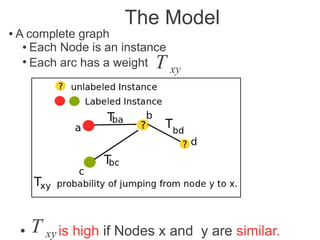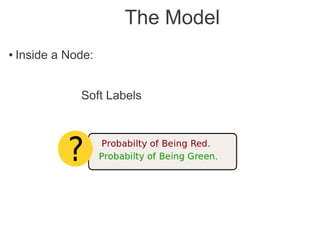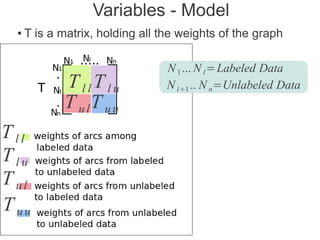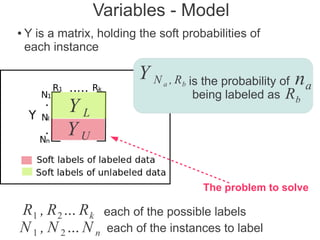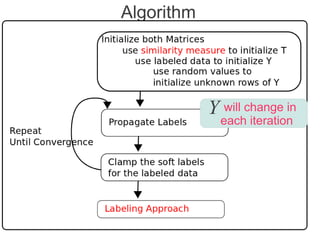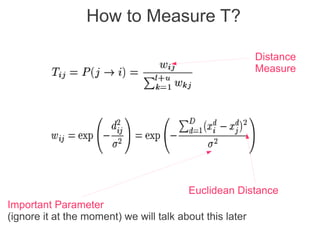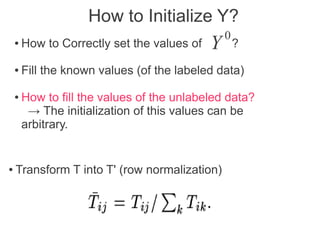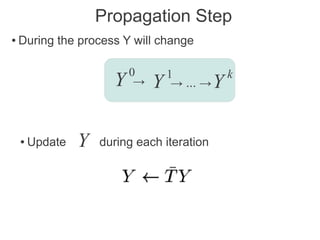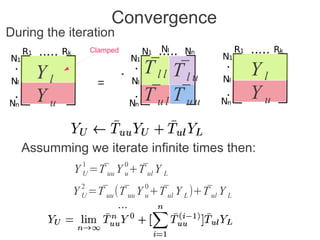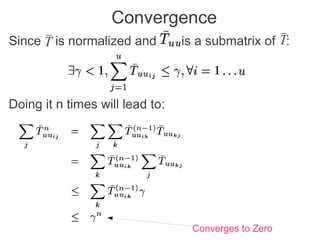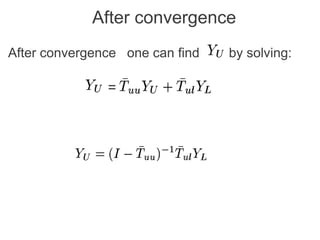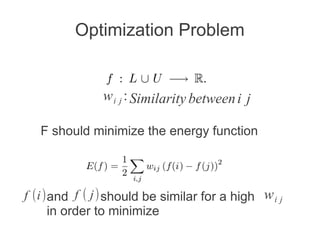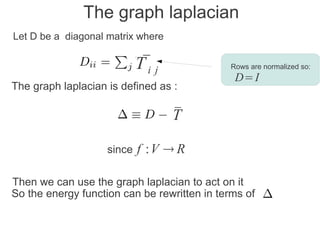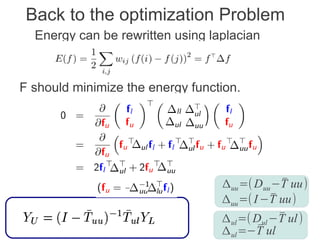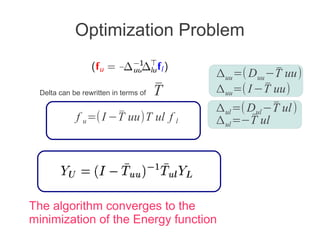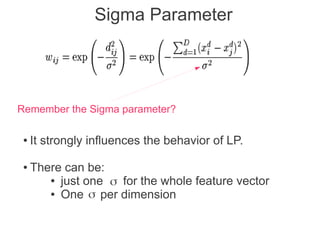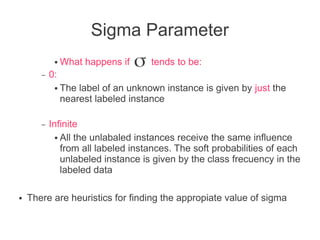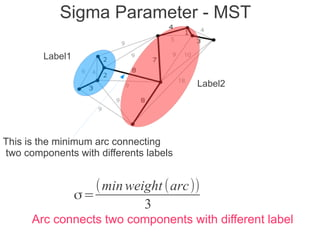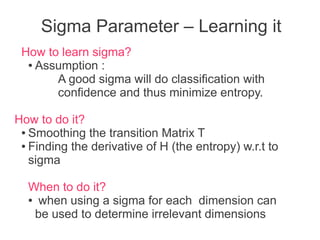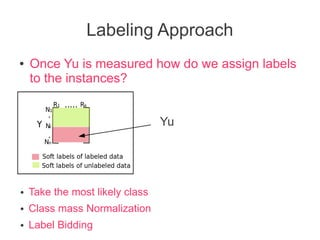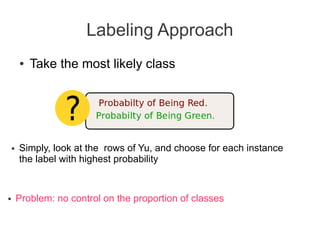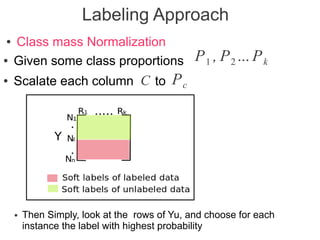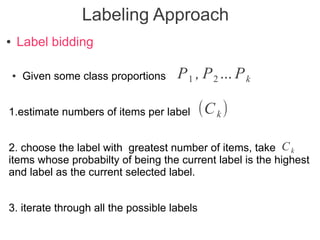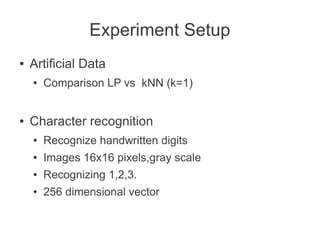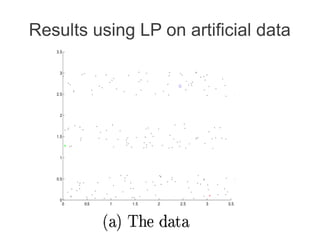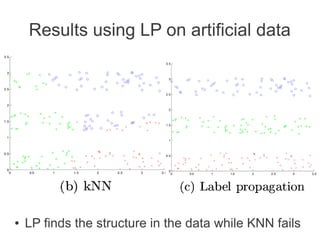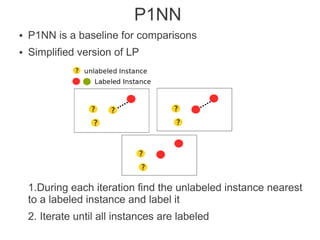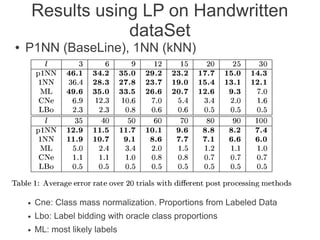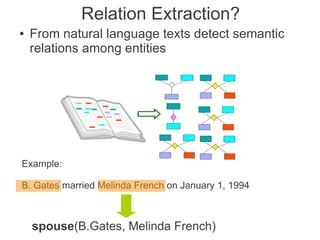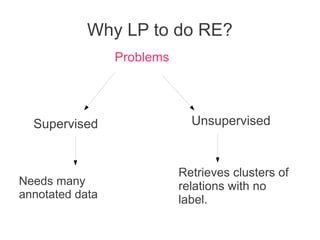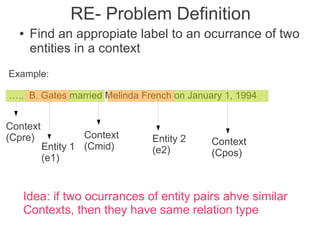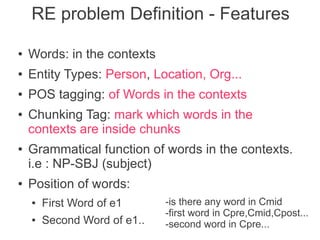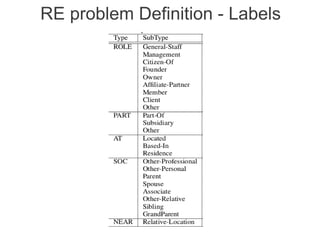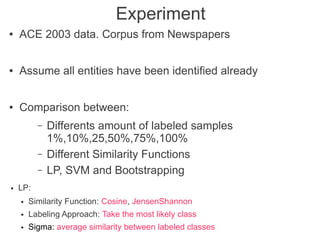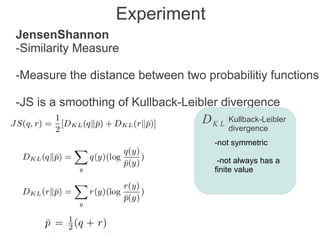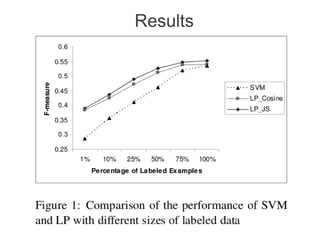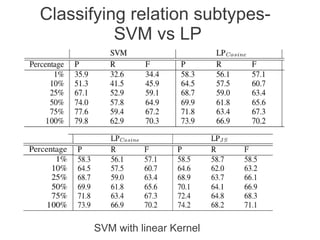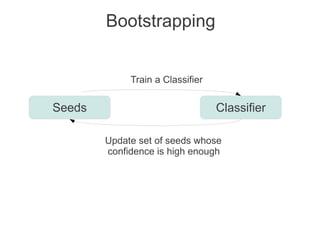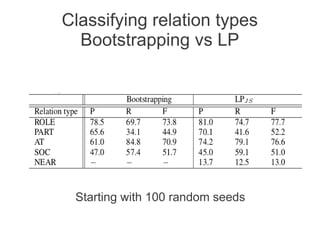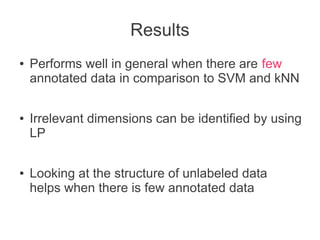1 of 46

### Label propagation - Semisupervised Learning with Applications to NLP

1. Label Propagation Seminar: Semi-supervised and unsupervised learning with Applications to NLP David Przybilla davida@coli.uni-saarland.de
2. Outline ● What is Label Propagation ● The Algorithm ● The motivation behind the algorithm ● Parameters of Label Propagation ● Relation Extraction with Label Propagation
3. Label Propagation ● Semi-supervised ● Shows good results when the amount of annotated data is low with respect to the supervised options ● Similar to kNN
4. K-Nearest Neighbors(KNN) ● Shares similar ideas with Label Propagation ● Label Propagation (LP) uses unlabeled instances during the process of finding out the labels
5. Idea of the Problem Similar near Unlabeled Instances should have similar Labels L=set of Labeled Instances U =set of Unlabeled Instances We want to find a function f such that:
6. The Model ● A complete graph ● Each Node is an instance ● Each arc has a weight T xy ● T xy is high if Nodes x and y are similar.
7. The Model ● Inside a Node: Soft Labels
8. Variables - Model ● T is a matrix, holding all the weights of the graph N 1 ... N l = Labeled Data TllTlu N l+1 .. N n=Unlabeled Data T u lT u u Tll Tlu T ul T uu
9. Variables - Model ● Y is a matrix, holding the soft probabilities of each instance YN a n , R b is the probability of a being labeled as R b YL YU The problem to solve R1 , R 2 ... R k each of the possible labels N 1 , N 2 ... N n each of the instances to label
10. Algorithm Y will change in each iteration
11. How to Measure T? Distance Measure Euclidean Distance Important Parameter (ignore it at the moment) we will talk about this later
12. How to Initialize Y? 0 ● How to Correctly set the values of Y ? ● Fill the known values (of the labeled data) ● How to fill the values of the unlabeled data? → The initialization of this values can be arbitrary. ● Transform T into T' (row normalization)
13. Propagation Step ● During the process Y will change 0 1 k Y → Y → ... → Y ● Update Y during each iteration
14. Convergence During the iteration Clamped Yl ̄ T l l T̄l u Yl = Yu T̄u l T̄ u u Yu Assumming we iterate infinite times then: 1 Y =T U ̄uu Y 0+ T ul Y L u ̄ 2 Y =T U ̄uu ( T̄uu Y 0 + T ul Y L )+T ul Y L u ̄ ̄ ...
15. Convergence ̄ Since T is normalized and ̄ is a submatrix of T: Doing it n times will lead to: Converges to Zero
16. After convergence After convergence one can find by solving: =
17. Optimization Problem w i j : Similarity between i j F should minimize the energy function f (i ) and f ( j) should be similar for a high w i j in order to minimize
18. The graph laplacian Let D be a diagonal matrix where T̄i j Rows are normalized so: D= I The graph laplacian is defined as : ̄ T since f :V → R Then we can use the graph laplacian to act on it So the energy function can be rewritten in terms of
19. Back to the optimization Problem Energy can be rewritten using laplacian F should minimize the energy function. ̄ Δuu =( D uu −T uu) ̄ Δuu =( I −T uu) ̄ Δ ul =( Dul − T ul ) ̄ Δ ul =−T ul
20. Optimization Problem ̄ Δuu =( D uu −T uu) Delta can be rewritten in terms of ̄ T ̄ Δ uu=( I − T uu) ̄ Δ ul =( Dul − T ul ) ̄ f u =( I −T uu)T ul f l ̄ Δ ul =−T ul The algorithm converges to the minimization of the Energy function
21. Sigma Parameter Remember the Sigma parameter? ● It strongly influences the behavior of LP. ● There can be: ● just one σ for the whole feature vector ● One σ per dimension
22. Sigma Parameter ● What happens if σ tends to be: – 0: ● The label of an unknown instance is given by just the nearest labeled instance – Infinite ● All the unlabaled instances receive the same influence from all labeled instances. The soft probabilities of each unlabeled instance is given by the class frecuency in the labeled data ● There are heuristics for finding the appropiate value of sigma
23. Sigma Parameter - MST Label1 Label2 This is the minimum arc connecting two components with differents labels (min weight (arc)) σ= 3 Arc connects two components with different label
24. Sigma Parameter – Learning it How to learn sigma? ● Assumption : A good sigma will do classification with confidence and thus minimize entropy. How to do it? ● Smoothing the transition Matrix T ● Finding the derivative of H (the entropy) w.r.t to sigma When to do it? ● when using a sigma for each dimension can be used to determine irrelevant dimensions
25. Labeling Approach ● Once Yu is measured how do we assign labels to the instances? Yu ● Take the most likely class ● Class mass Normalization ● Label Bidding
26. Labeling Approach ● Take the most likely class ● Simply, look at the rows of Yu, and choose for each instance the label with highest probability ● Problem: no control on the proportion of classes
27. Labeling Approach ● Class mass Normalization ● Given some class proportions P 1 , P 2 ... P k ● Scalate each column C to Pc ● Then Simply, look at the rows of Yu, and choose for each instance the label with highest probability
28. Labeling Approach ● Label bidding ● Given some class proportions P 1 , P 2 ... P k 1.estimate numbers of items per label (C k ) 2. choose the label with greatest number of items, take C k items whose probabilty of being the current label is the highest and label as the current selected label. 3. iterate through all the possible labels
29. Experiment Setup ● Artificial Data ● Comparison LP vs kNN (k=1) ● Character recognition ● Recognize handwritten digits ● Images 16x16 pixels,gray scale ● Recognizing 1,2,3. ● 256 dimensional vector
30. Results using LP on artificial data
31. Results using LP on artificial data ● LP finds the structure in the data while KNN fails
32. P1NN ● P1NN is a baseline for comparisons ● Simplified version of LP 1.During each iteration find the unlabeled instance nearest to a labeled instance and label it 2. Iterate until all instances are labeled
33. Results using LP on Handwritten dataSet ● P1NN (BaseLine), 1NN (kNN) ● Cne: Class mass normalization. Proportions from Labeled Data ● Lbo: Label bidding with oracle class proportions ● ML: most likely labels
34. Relation Extraction? ● From natural language texts detect semantic relations among entities Example: B. Gates married Melinda French on January 1, 1994 spouse(B.Gates, Melinda French)
35. Why LP to do RE? Problems Supervised Unsupervised Retrieves clusters of Needs many relations with no annotated data label.
36. RE- Problem Definition ● Find an appropiate label to an ocurrance of two entities in a context Example: ….. B. Gates married Melinda French on January 1, 1994 Context (Cpre) Context Entity 2 Entity 1 (Cmid) Context (e2) (Cpos) (e1) Idea: if two ocurrances of entity pairs ahve similar Contexts, then they have same relation type
37. RE problem Definition - Features ● Words: in the contexts ● Entity Types: Person, Location, Org... ● POS tagging: of Words in the contexts ● Chunking Tag: mark which words in the contexts are inside chunks ● Grammatical function of words in the contexts. i.e : NP-SBJ (subject) ● Position of words: ● First Word of e1 -is there any word in Cmid -first word in Cpre,Cmid,Cpost... ● Second Word of e1.. -second word in Cpre...
38. RE problem Definition - Labels
39. Experiment ● ACE 2003 data. Corpus from Newspapers ● Assume all entities have been identified already ● Comparison between: – Differents amount of labeled samples 1%,10%,25,50%,75%,100% – Different Similarity Functions – LP, SVM and Bootstrapping ● LP: ● Similarity Function: Cosine, JensenShannon ● Labeling Approach: Take the most likely class ● Sigma: average similarity between labeled classes
40. Experiment JensenShannon -Similarity Measure -Measure the distance between two probabilitiy functions -JS is a smoothing of Kullback-Leibler divergence DK L Kullback-Leibler divergence -not symmetric -not always has a finite value
41. Results
42. Classifying relation subtypes- SVM vs LP SVM with linear Kernel
43. Bootstrapping Train a Classifier Seeds Classifier Update set of seeds whose confidence is high enough
44. Classifying relation types Bootstrapping vs LP Starting with 100 random seeds
45. Results ● Performs well in general when there are few annotated data in comparison to SVM and kNN ● Irrelevant dimensions can be identified by using LP ● Looking at the structure of unlabeled data helps when there is few annotated data
46. Thank you